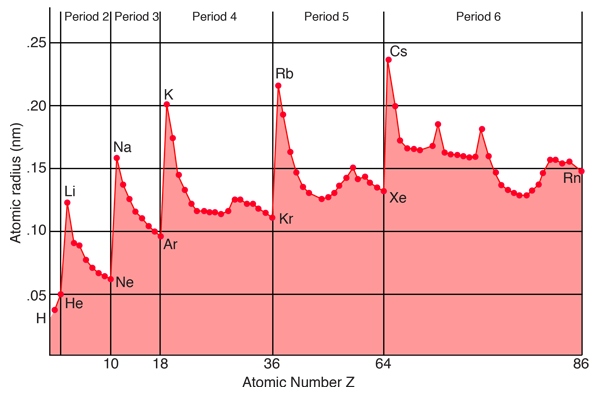In specifying values for the radius of an atom, one must keep in mind the fact that atoms are not hard spheres, and the electron distribution in the outer part of the atom does not have a sharp cutoff radius. You could characterize the radius of the atom as a limiting radius where a certain percentage of the electron charge will be found. The illustration above is a plot of "covalent radii" (from Ebbing) which are determined by measuring the bond lengths in the molecules of chemical compounds. Another way to determine characteristic radii is to measure ionic radii in crystals using x-ray diffraction. If the crystalline composition is such that the ions can be considered to be in contact with each other, and you can determine the lattice spacing from x-ray diffraction, then you can imply the ionic radius. As might be expected, the ionic radius of negative ions is slightly larger than the covalent radius since they have extra electronic charge, and that of positive ions is slightly smaller.

The atomic radius is one of the parameters used in quantifying chemical bonds.

Index

Chemical bond concepts

Reference
Ebbing
Ch 9

 HyperPhysics***** Chemistry R Nave
Go Back